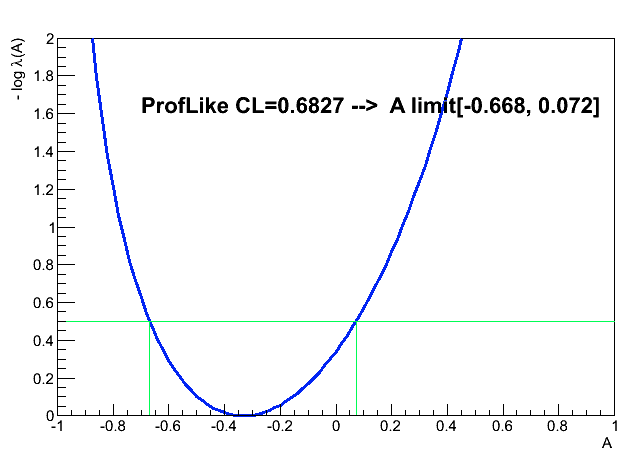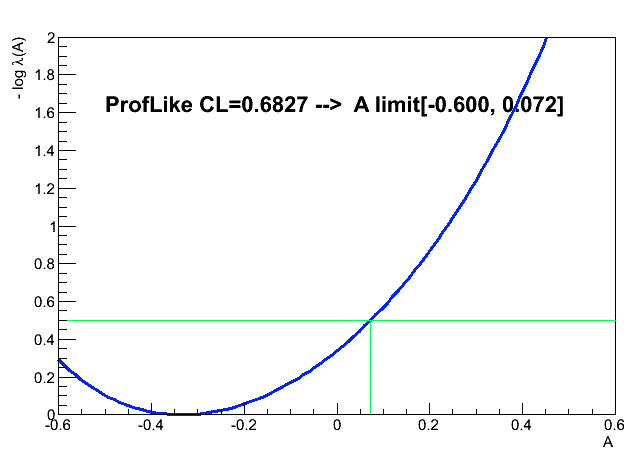# CL interval not accounting for domain of parOfInterest

Hi,
I’m exploring use of the RooStat package to extract asymmetry for a pair of measured yields (the actual problem is extraction of the spin asymmetry, but this simplification captures the essence of the math).

The RooStats tutorials were very helpful and I got a woking test-bed code for my simplified task.
But I do not understand how the LikelihoodInterval class takes in to account the constrain on the domain of parameter of interest ?
I’m seeking advice how to overcome this issue.

Below is a short synopsis of my simplified problem, attached code works but produces result I question.

Definition of the problem:
I measure 2 independent yields n1, n2 in an experiment. Analytically I’d reconstruct the quantity: A= (n1-n2)/(n1+n2)
From independent source (physics interpretation of A) I know magnitude of A must be below some value Amax, lets say Amax=1.

In oder to apply the likelihood method using RooStats package this problem can be expressed as follows:

There are 2 parameters of the underlying model: N0,A, out of which
A is parameter of interest, N0 is the nuisance parameter.

Let variables mu1, mu2 are predictions of 2 yields based on the model:
mu1=N0(1+A)
mu2=N0(1-A)

The probability of measuring yield ‘n’ given model prediction ‘mu’ is given by the Poisson distribution f( n | mu).

The total likelihood of measuring a pair (n1,n2) is
Ltot(A,N0)=f(n1 | mu1) * f (n1 |mu2) * H( Amax - |A|)
where H(x) is the Heaviside step function.

The attached macro jan_2spinAsy_constrain.C does the computation and fig 1 shows the outcome for n1=2, n2=4 (yes, very low stats), Amax=1, and CL=0.6827 the output is: limit[-0.668, 0.072].Now, I repeat extraction of A from the same pair of input yields (n1=2, n2=4 ) but under different assumption fabs(A)<0.6 (say a theorist called me and said if A >0.6 the World would never exist)

The result of ProfileLikelihoodCalculator using narrower domain for A=[-0.6,0.6] are different only on the lower limit, see Fig. 2. The upper limit of 0.072 stays unchanged.Here is the issue:
According to CL definition, the fraction of the area below lambda(A) over confidence limit should be equal to CL. If I trim the domain of A it should be taken in to account and the upper confidence range for the same CL should move up.

Is there a way I can ask ProfileLikelihoodCalculator to include constrain |A|< Amax while returning :: GetInterval() given CL ?

Thanks
Jan
jan_2spinAsy_constrain.C (2.52 KB)

Hi ,

I tried to resolve this issue by skipping the last several steps of RooStats. From RooStats, via function GetLikelihoodRatio(),http://root.cern.ch/root/html/RooStats__LikelihoodInterval.html#RooStats__LikelihoodInterval:GetLikelihoodRatio, we can get the function of negative logarithm of the profile likelihood ratio (normalize L to let the maximum to be 1, L/L_max, upper plot in attached fig1). Instead of getting confidence interval from RooStats, we can just leave it with this function, -ln(L/L_max).

1. convert -ln(L/L_max) to L/L_max, lower plot in attached fig1 ;
2. find the interval with give confidence level
2.1) go down from the peak step by step to get indivial interval,
2.2) compute the ratio of each interval and the full domain,
2.3) compare the ratio with give confidence level to find our purposed interval.

From the attach figure, we can see that the interval from this method is narrower than interval from RoosStats. This is because of that after the tail is cut off, the denominator is reduced more than the numerator in computing the ratio, 2.2).

Attached .zip are the macros I have used to implement this method.

root -l spin2Asy_constraint.C

There is an issue for this method. When convert the -ln(L/L_max) to L/L_max, I have used medium histogram with fixed 100 bins by using TF1::GetHistogram(). So, the precision is limited by this fixed 100 bins. Is there a way to control the number of bins ?

Thanks,
Jinlong

spin2Asy_getInterval_constraint.zip (2.7 KB)